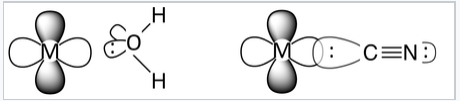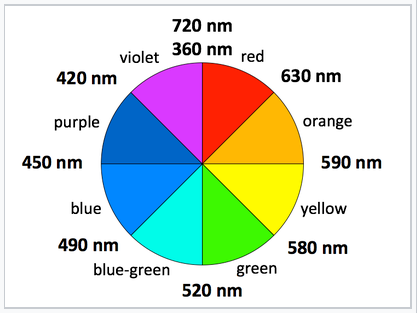# 4.6: Spectrochemical Series

$$\newcommand{\vecs}{\overset { \rightharpoonup} {\mathbf{#1}} }$$ $$\newcommand{\vecd}{\overset{-\!-\!\rightharpoonup}{\vphantom{a}\smash {#1}}}$$$$\newcommand{\id}{\mathrm{id}}$$ $$\newcommand{\Span}{\mathrm{span}}$$ $$\newcommand{\kernel}{\mathrm{null}\,}$$ $$\newcommand{\range}{\mathrm{range}\,}$$ $$\newcommand{\RealPart}{\mathrm{Re}}$$ $$\newcommand{\ImaginaryPart}{\mathrm{Im}}$$ $$\newcommand{\Argument}{\mathrm{Arg}}$$ $$\newcommand{\norm}{\| #1 \|}$$ $$\newcommand{\inner}{\langle #1, #2 \rangle}$$ $$\newcommand{\Span}{\mathrm{span}}$$ $$\newcommand{\id}{\mathrm{id}}$$ $$\newcommand{\Span}{\mathrm{span}}$$ $$\newcommand{\kernel}{\mathrm{null}\,}$$ $$\newcommand{\range}{\mathrm{range}\,}$$ $$\newcommand{\RealPart}{\mathrm{Re}}$$ $$\newcommand{\ImaginaryPart}{\mathrm{Im}}$$ $$\newcommand{\Argument}{\mathrm{Arg}}$$ $$\newcommand{\norm}{\| #1 \|}$$ $$\newcommand{\inner}{\langle #1, #2 \rangle}$$ $$\newcommand{\Span}{\mathrm{span}}$$

Strong and weak field ligands. The spectrochemical series ranks ligands according the energy difference ΔO between the t2g and eg orbitals in their octahedral complexes. This energy difference is measured in the spectral transition between these levels, which often lies in the visible part of the spectrum and is responsible for the colors of complexes with partially filled d-orbitals. Ligands that produce a large splitting are called strong field ligands, and those that produce a small splitting are called weak field ligands.An abbreviated spectrochemical series is:

Weak field I- < Br- < Cl- < NO3- < F- < OH- < H2O < Pyridine < NH3 < NO2- < CN- < CO Strong field

## Orbital overlap

Referring to the molecular orbital diagram above, we see that the splitting between d-electron levels reflects the antibonding interaction between the eg metal orbitals and the ligands. Thus, we expect ligand field strength to correlate with metal-ligand orbital overlap. Ligands that bind through very electronegative atoms such as O and halogens are thus expected to be weak field, and ligands that bind through C or P are typically strong field. Ligands that bind through N are intermediate in strength. Another way to put this is that hard bases tend to be weak field ligands and soft bases are strong field ligands.Water is a weak field ligand. The electronegative O atom is strongly electron-withdrawing, so there is poor orbital overlap between the electron pair on O and a metal d-orbital. The more electropositive C atom in the strong field ligand CN- allows better orbital overlap and sharing of the electron pair. Note that CN- typically coordinates metal ions through the C atom rather than the N atom.Cobalt (II) complexes have different colors depending on the nature of the ligand. In crystals of the red compound cobalt(II) nitrate dihydrate, each cobalt ion is coordinated by six water molecules. The [Co(H2O)6]2+ cations and NO3- anions crystallize to make a salt. When the complex is dissolved in water, Co(II) retains its coordination shell of six water molecules and the solution has the same red color as the crystal.

## Energy units

Energy can be calculated in a number of ways and it is useful to try to relate the splitting energy ΔO to more familiar quantities like bond energies.

$E=h\nu=h\frac{c}{\lambda} = hc{\tilde{\nu}}$

Here ν is the frequency of the electromagnetic radiation, h is Planck's constant (6.626x10-34 J*s), and c is the speed of light. $${\tilde{\nu}}$$ is called the "wavenumber" and is the inverse of the wavelength, usually measured in cm-1. Energy gaps are often expressed by spectroscopists in terms of wavenumbers.

For example, a red photon has a wavelength of about 620 nm and a wavenumber of about 16,000 cm-1. In other energy units, the same red photon has an energy of 2.0 eV (1 eV = 1240 nm) or 193 kJ/mol (1 eV = 96.5 kJ/mol). If we compare this to the dissociation energy of a carbon-carbon single bond (350 kJ/mol), we see that the C-C bond has about twice the energy of a red photon. We would need an ultraviolet photon (E > 350 kJ/mol = 3.6 eV = 345 nm = 29,000cm-1) to break a C-C bond.

We will see that ΔO varies widely for transition metal complexes, from near-infrared to ultraviolet wavelengths. Thus the energy difference between the t2g and eg orbitals can range between the energy of a rather weak to a rather strong covalent bond.

ΔO depends on both the metal and the ligand. We can learn something about trends in ΔO by comparing a series of d6 metal complexes

Complex ΔO (cm-1)
[Co(H2O)6]2+ 9,300
[Co(H2O)6]3+ 18,200
[Co(CN)6]3- 33,500
[Rh(H2O)6]3+ 27,000
[Rh(CN)6]3- 45,500
• Co3+ complexes have larger ΔO than Co2+ complexes with the same ligand. This reflects the electrostatic nature of the crystal field splitting.
• Rh3+ complexes have larger ΔO than Co3+ complexes. In general, elements in the 2nd and 3rd transition series (the 4d and 5d elements) have larger splitting than those in the 3d series.

For a given metal in one oxidation state (e.g., Co3+), the trend in ΔO follows the spectrochemical series. Thus ΔO is larger for [Co(CN)6]3-, which contains the strong field CN- ligand, than it is for [Co(H2O)6]3+ with the weak field ligand H2O.

The 4d and 5d elements are similar in their size and their chemistry. In comparing ΔO values for complexes in the 3d, 4d, and 5d series (e.g., comparing elements in the triads Co,Rh,Ir or Fe,Ru,Os), we always find 3d << 4d ≲ 5d. This trend reflects the spatial extent of the d-orbitals and thus their overlap with ligand orbitals. The 3d orbitals are smaller, and they are less effective in bonding than the 4d or 5d. The 4d and 5d orbitals are similar to each other because of the lanthanide contraction. At the beginning of the 5d series (between 56Ba and 72Hf) are the fourteen lanthanide elements (57La - 71Lu).

Although the valence orbitals of the 5d elements are in a higher principal quantum shell than those of the 4d elements, the addition of 14 protons to the nucleus in crossing the lanthanide series contracts the sizes of the atomic orbitals. The important result is that the valence orbitals of the 4d and 5d elements have similar sizes and thus the elements resemble each other in their chemistry much more than they resemble their cousins in the 3d series. For example, the chemistry of Ru is very similar to that of Os, as illustrated below, but quite different from that of Fe.Both Os and Ru form volatile, molecular tetroxides MO4. OsO4 is used in epoxidation reactions and as a stain in electron microscopy. In contrast, the highest binary oxide of iron is Fe2O3.

## Colors of transition metal complexes

A simple, qualitative way to see the relative crystal field splitting energy, ΔO, is to observe the color of a transition metal complex. The higher the energy of the absorbed photon, the larger the energy gap. However, the color a complex absorbs is complementary to the color it appears (i.e., the color of light it reflects), which is opposite the absorbed color on the color wheel.Complementary colors are across the color wheel from each other

Examples: (all d7 Co2+ complexes)

[Co(H2O)6]2+ looks purple in its salts and in concentrated solution because it absorbs in the green range.

[Co(NH3)6]2+ is straw-colored because it absorbs in the blue range.

[Co(CN)6]4-, looks red, absorbs in the violet and ultra-violet part of the spectrum. This is consistent with the idea that CN- is a stronger field ligand than NH3, because the energy of a UV photon is higher than that of a red-orange photon.

This method is applicable to most transition metal complexes, as the majority of them absorb somewhere in the visible range (400-700 nm = 25,000 to 14,300 cm-1), or have UV transitions that tail into the visible, making them appear yellow; however there are complexes such as [Rh(CN)6]3- that appear colorless because their d-d transitions are in the ultraviolet. Other complexes such as [Mn(H2O)]62+ are weakly colored because their d-d transitions involve a change in the spin state of the complex.

4.6: Spectrochemical Series is shared under a CC BY-SA license and was authored, remixed, and/or curated by LibreTexts.